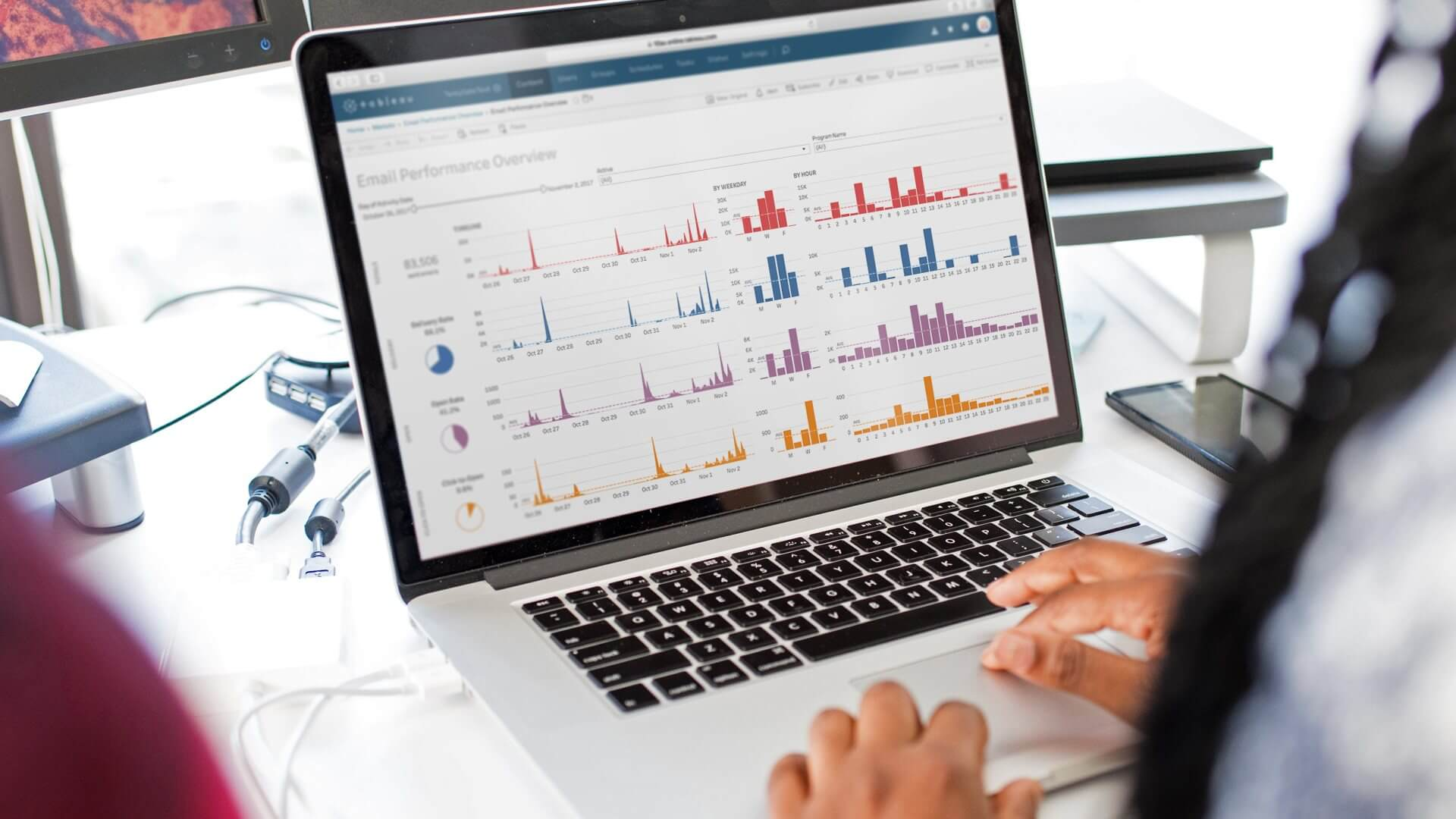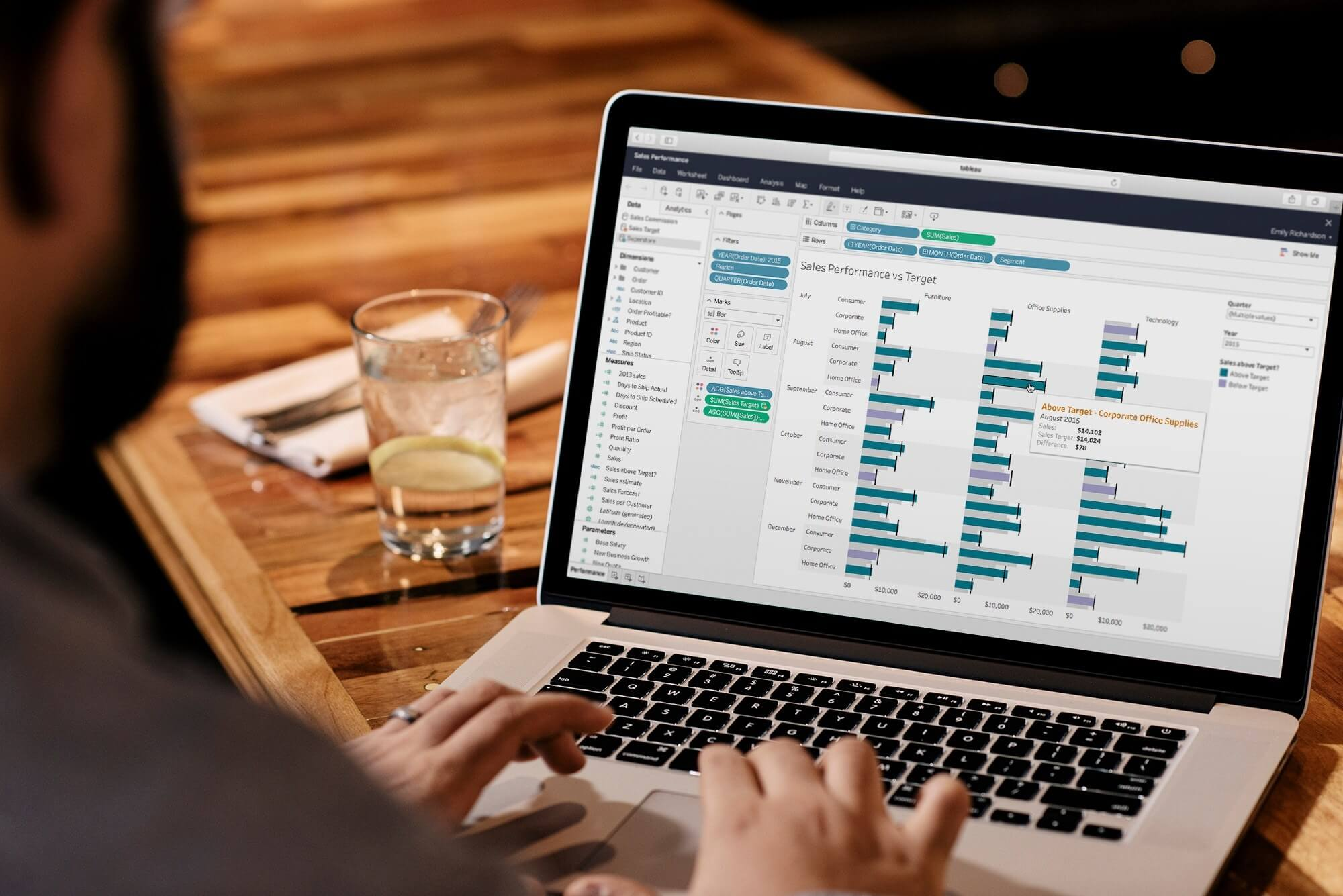Tutorial

# Mapping Real Time Flights (Advanced)July 1, 2018

## 1. The Data

The best would be to read the beginners' version of this blog post to learn which fields are necessary and sufficient to build such a viz. Have a flight to catch? Here's a quick summary:

• 2 datetime fields, in this example: Departure_Datetime and Arrival_Datetime
• 2 points geocoded by latitude and longitude, here: Departure_Lat, Departure_Lon, Arrival_Lat, Arrival_Lon

Second you need an image of a plane (or train, or car) ! I chose the simple ✈ icon below. You could choose any other image, but the nose of the plane has to be towards the right! Name it plane.png.## 3. Generate 36 Icons Thanks to Python!

Then, you want your little airplane to fly in all directions, all over the planet! Thus, you'll need to make 36 images of the plane, each one rotated by 10°. But this is tedious and I am lazy so I used a little code to make this quickly ! Do you know Python? This is one of the easiest programming languages I know. If time allows, you'll definitely benefit from learning it!

Just download Python 2.7, and open a command line Windows + R on Windows then ‘cmd’ enter.

Type in the following command:

pip install pil

This command should install a python library for image processing. Then create a new file with a .py extension. Open it with your notepad for instance and copy-paste the following code:

from PIL import Image
src_im = Image.open("plane.png")
im = src_im.convert('RGBA')
for i in range(36):
rot = im.rotate(i*10)
rot.save(str(i*10).zfill(3)+"plane.png")

See? I have written all the code for you, save your new python file with this nice code and that’s it! Time to run it!

If you want to run the code, simply open your command line like before and run:

Python yourscript.py

It should create for you 36 images of your plane each time rotated by 10° !!! Et voilà! It wasn’t hard to code a little, was it? Now, just copy your shapes in a new folder in your Tableau Repository's Shapes file.

## 4. Be Ready for Some Geocoding

Lines on a map in Tableau are great but they do not reflect the curvature of the earth, so we need to approximate it. There is all you need to know about bearings, geo-coordinates and angles on this website. You can also read this Wikipedia page.

So first we need to calculate our latitudes and longitudes for departures and arrivals in radians rather than in degrees, because we are going to use a lot of SIN() and COS() and they only work in radians. Those are the fields you will see later in formulas as Dep Lat Rad, Dep Lat Long, Arr Lat Rad et Arr Lat Long.

Then, we will need to create the following calculated fields in order to obtained the curved routes:
h = sin²(Δφ/2) + cos φ1 ⋅ cos φ2 ⋅ sin²(Δλ/2)
δ = d/R = 2 ⋅ atan2( √h, √(1− h) )
A = sin((1−f)⋅δ) / sin δ
B = sin(f⋅δ) / sin δ
x = A ⋅ cos φ1 ⋅ cos λ1 + B ⋅ cos φ2 ⋅ cos λ2
y = A ⋅ cos φ1 ⋅ sin λ1 + B ⋅ cos φ2 ⋅ sin λ2
z = A ⋅ sin φ1 + B ⋅ sin φ2
φi = atan2(z, √x² + y²)
λi = atan2(y, x)

It might be a bit intimidating displayed like this, but don’t worry I have broken it down for you and taken screenshots of my Calculated Fields in the next section.

a. Calculate how much of the flight has already been done based on your departure and arrival datetimes:

• MAX(MIN(DATEDIFF('minute',NOW(),[Departure Datetime])/DATEDIFF('minute', [Arrival Datetime],[Departure Datetime]),1),0)b. Calculate the haversine formula with your latitudes and longitudes for departure and arrival (in radians with the function RADIANS()):c. Calculate the angular distance between the departure point and the arrival point:

• 2 * ATAN2 (SQRT([haversine(d/r)]), SQRT(1 - [haversine(d/r)]))d. From here, you just need to recalculate the coefficients of the formula:

• SIN((1-[Percent Flight])*[angular Distance]) / SIN([angular Distance])• SIN([Percent Flight] * [angular Distance]) / SIN([angular Distance])• [A] * SIN([Dep Lat Rad]) + [B] * SIN([Arr Lat Rad])e. Almost done! You can now calculate the estimated geo coordinates of the aircraft as follows:

• ATAN2([z], SQRT([x]^2 + [y]^2))

WARNING, the result is in radians, don’t forget to use DEGREES() to get a result Tableau can understand.• ATAN2([y], [x])f. And you can calculate its orientation as follows:• RIGHT("00"+REPLACE(STR(FLOOR(IIF([aircraft orientation]If you have enough planes, they should statistically display any orientation between 000 and 350. Therefore, you can assign the new shapes to the field “approx. aircraft orientation”. The shapes should automatically be perfectly assigned to each plane since Tableau assigns them alphabetically.

You now need to drag and drop your last 2 calculated fields “aircraft lat” to rows and “aircraft lon” to columns.Last but not least, if your viz is showing real time data, use a filter to only display the planes for which departuredate now(). This will leave only the planes currently in the air right now!

And there you go! A Flightradar24 like viz in Tableau! If you want, you can try and take this approach a little bit further to get some trails behind the planes!

#### More articles###### Data analysis - Six steps to better business decisions
September 26, 2019April 3, 2019